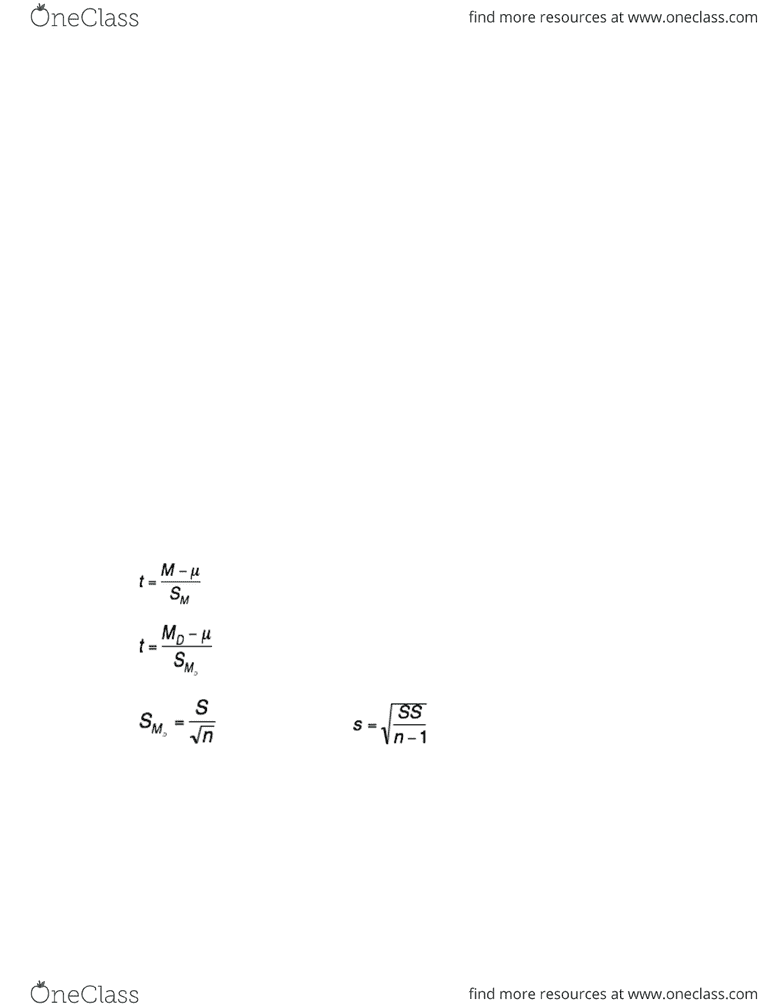Class Notes (1,100,000)
CA (620,000)
Carleton (20,000)
PSYC (3,000)
PSYC 2002 (100)
Lecture 7

# PSYC 2002 Lecture Notes - Lecture 7: Repeated Measures Design, Speed Reading, Null Hypothesis

Department
Psychology
Course Code
PSYC 2002
Professor
Angel Mackenzie
Lecture
7

This preview shows pages 1-3. to view the full 10 pages of the document.Thursday, February 25th, 2016
PSYC 2002 – t-test (independent)
Summary from last class:
- Review of concepts
- Single sample t-test
oDegrees of freedom
ot-distributions
t distribution table
oHypothesis testing w/ t-tests
Outline: t-tests
- Confidence intervals & effect size for single-sample t
- Assumptions of single sample t-test
- Related samples t-test
- Independent samples-t:
oLimitations of single-sample t-test
oDevelopment of general formula for independent sample t-test
Readings:
- Related samples t-test
oNH Chapter 9 (The single-sample t-test & paired-samples t-test)
- Independent samples t-test
oNH Chapter 10 (The independent samples t-test)
Confidence intervals using t:
- Confidence intervals using t are similar in form to those used w/ Z
- General CI formula: CI limits = statistic ± (α value)(SE)
Confidence intervals using t: Single sample t-test
- CI for Z-test:
CI limits = M + (Z)(σM)
Where M = sample mean; Z = critical point based on α (e.g., 1.96)
- CI for t-test:
CI limits M + (t)(sM)
Where M = sample mean; t = tcritical associated w/ degrees of freedom
for sample size n; sM = standard error of mean
- Remember: CI = alternative to hypothesis testing, but we’re also testing it against null
hypothesis
- Our confidence level (e.g., 95%) determines how confident we are that mean of true
population (according to null hypothesis) falls within CI limits
- If sample mean does NOT fall limits, it’s a low probability event (e.g., less than 5%
probability)

Only pages 1-3 are available for preview. Some parts have been intentionally blurred.oM likely belongs to another population
oSimilar to rejecting null hypothesis
Effect size for single sample t-test:
- Cohen’s d for z-test:
- Cohen’s d for t-test:
d = M - µ
s
- Same formula for effect size, but using sample SD instead of population SD
Assumptions of single sample t-test:
1. Values in sample must be independent observations
oIf value of each observation cannot be predicted from value of another
observation, then observations are independent
2. Population sampled must be normal
oEsp true if sample size is small; for samples larger than n = 30, violations of
normality assumption have little effect on results
3. Dependent variable measured on ratio or interval scale
Limitations of the single-sample t-test:
- Single sample t-test used to test hypothesis about single sample & its relationship to
population (M vs. μ)
- Researchers often compare 2 samples: is treatment A better than treatment B or is
performance in condition 1 better than condition 2?
Utility of single-sample t-test:
- Single-sample t-tests aren’t used frequently in research
- Why? – typically researchers are more interested in comparing means obtained from
samples than in comparing sample mean to population mean
oRelated samples t-test
oIndependent samples test
Related samples t-test:
- Important research design involves testing same individuals in 2 diff conditions
oRepeated measures design (aka within-subjects design)
- Similarly, individuals can be matched on some characteristic, then tested in 2 diff
conditions
oMatched design (aka paired design)
- Both designs: some sort of “relationship” b/w 2 samples
- Both types of related samples (repeated measure & matched) designs:
oControlling or limiting variability among participants

Only pages 1-3 are available for preview. Some parts have been intentionally blurred.oMakes them more sensitive to effects of independent variable than designs in
which 2 independent groups are compared
- In both cases, variation on t-test formula called related samples t-test (aka t-test
dependent means or matched/paired samples t-test) used
- Related samples t-test differs from single sample t-test in 2 ways:
1. Uses difference scores
2. Assumes (null hypothesis): µ = 0 (i.e., µ1 - µ2 = 0)
oMean of distribution of differences b/w 2 related sample groups is 0
Related samples t-test: Difference scores (*chart in lecture slide*)
- Mean difference score = 12/6 = +2
oNot equal to 0 – does this suggest there’s a difference b/w samples?
- Sample difference scores related to population of difference scores
oDoes our finding of +2 indicate that there’s a true difference b/w these groups in
population?
- In before-after situations (repeated-measures), NH suggest using after – before
subtraction order to determine difference score; this changes sign of difference score
oAn increase (i.e., before small, after big) = positive difference score
oA decrease (i.e., before big, after small) = negative difference score
oE.g., post-test minus pre-test score: how did you score on 2nd test compared to 1st?
Better (positive difference)?
Worse (negative difference)?
General formula for related samples t-test:
- Begin w/ standard t-test formula:
- By substitution, related samples formula:
(D at the bottom of M)
- Where standard error of mean differences (SM ) is:
Remember:
-(SM ) calculated same way as s for single-sample t
- Degrees of freedom (denominator for S) defined as n-1, number of difference scores -1
oDiffers from single-sample t as n refers to number of participants, not number of
scores
Practice:
D
D
###### You're Reading a Preview

Unlock to view full version